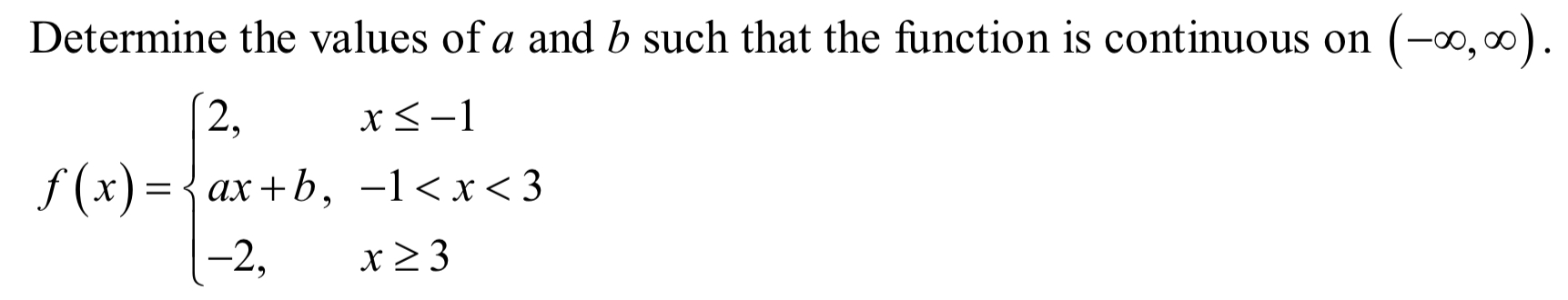# Determine the values of a and b such that the function is continuous on (-00, oof(x)-ax+b, -l

Question
1 viewshelp_outlineImage TranscriptioncloseDetermine the values of a and b such that the function is continuous on (-00, oo f(x)-ax+b, -l
check_circle

Step 1

We have to find value of a and b so that function is continous is given interval  (-inf,inf)

Function  is given below:

Step 2

For function to be continous at x=a , following condition should hold  .

Condition is given below:

Step 3

Now look at question we have two points where we can check our continuity .

x=-1

x=3

First check at x=-1 . we will check left ha...

### Want to see the full answer?

See Solution

#### Want to see this answer and more?

Solutions are written by subject experts who are available 24/7. Questions are typically answered within 1 hour.*

See Solution
*Response times may vary by subject and question.
Tagged in

### Continuity